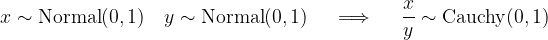﻿CauchyDistribution Class# CauchyDistribution Class

Represents a Cauchy distribution.Inheritance Hierarchy

Namespace:  Meta.Numerics.Statistics.Distributions
Assembly:  Meta.Numerics (in Meta.Numerics.dll) Version: 4.1.4Syntax
`public sealed class CauchyDistribution : ContinuousDistribution`

The CauchyDistribution type exposes the following members.Constructors
NameDescriptionCauchyDistribution
Initializes a new standard Cauchy distribution.CauchyDistribution(Double, Double)
Initializes a new Cauchy distribution.
TopProperties
NameDescriptionExcessKurtosis
Gets the excess kurtosis of the distribution.
(Inherited from UnivariateDistribution.)FullWithAtHalfMaximum
Gets the full width at half maximum (FWHM) of the Cauchy distribution.Mean
Gets the mean of the distribution.
(Overrides UnivariateDistributionMean.)Median
Gets the median of the distribution.
(Overrides ContinuousDistributionMedian.)Skewness
Gets the skewness of the distribution.
(Inherited from UnivariateDistribution.)StandardDeviation
Gets the standard deviation of the distribution.
(Inherited from UnivariateDistribution.)Support
Gets the interval over which the distribution is non-vanishing.
(Inherited from ContinuousDistribution.)Variance
Gets the variance of the distribution.
(Overrides UnivariateDistributionVariance.)
TopMethods
NameDescriptionCentralMoment
Computes a central moment of the distribution.
(Overrides ContinuousDistributionCentralMoment(Int32).)Cumulant
Computes a cumulant of the distribution.
(Overrides UnivariateDistributionCumulant(Int32).)Equals
Determines whether the specified object is equal to the current object.
(Inherited from Object.)ExpectationValue
Computes the expectation value of the given function.
(Inherited from ContinuousDistribution.)GetHashCode
Serves as the default hash function.
(Inherited from Object.)GetRandomValue
Generates a random variate.
(Overrides ContinuousDistributionGetRandomValue(Random).)GetRandomValues
Generates the given number of random variates.
(Inherited from ContinuousDistribution.)GetType
Gets the Type of the current instance.
(Inherited from Object.)Hazard
Computes the hazard function.
(Inherited from ContinuousDistribution.)InverseLeftProbability
Returns the point at which the cumulative distribution function attains a given value.
(Overrides ContinuousDistributionInverseLeftProbability(Double).)InverseRightProbability
Returns the point at which the right probability function attains the given value.
(Overrides ContinuousDistributionInverseRightProbability(Double).)LeftProbability
Returns the cumulative probability to the left of (below) the given point.
(Overrides ContinuousDistributionLeftProbability(Double).)ProbabilityDensity
Returns the probability density at the given point.
(Overrides ContinuousDistributionProbabilityDensity(Double).)RawMoment
Computes a raw moment of the distribution.
(Overrides ContinuousDistributionRawMoment(Int32).)RightProbability
Returns the cumulative probability to the right of (above) the given point.
(Overrides ContinuousDistributionRightProbability(Double).)ToString
Returns a string that represents the current object.
(Inherited from Object.)
TopRemarks

In physical applications, the Cauchy distribution is usually called a Lorentz distribution. It models the shape of a spectral line.

The ratio of two normally distributed quantities has a Cauchy distribution.The Cauchy distribution has "fat tails". In fact, it falls off at the minimum possible rate consistent with having a convergent probability integral. For this same reason, none of its moments (above the zeroth) are defined.See Also